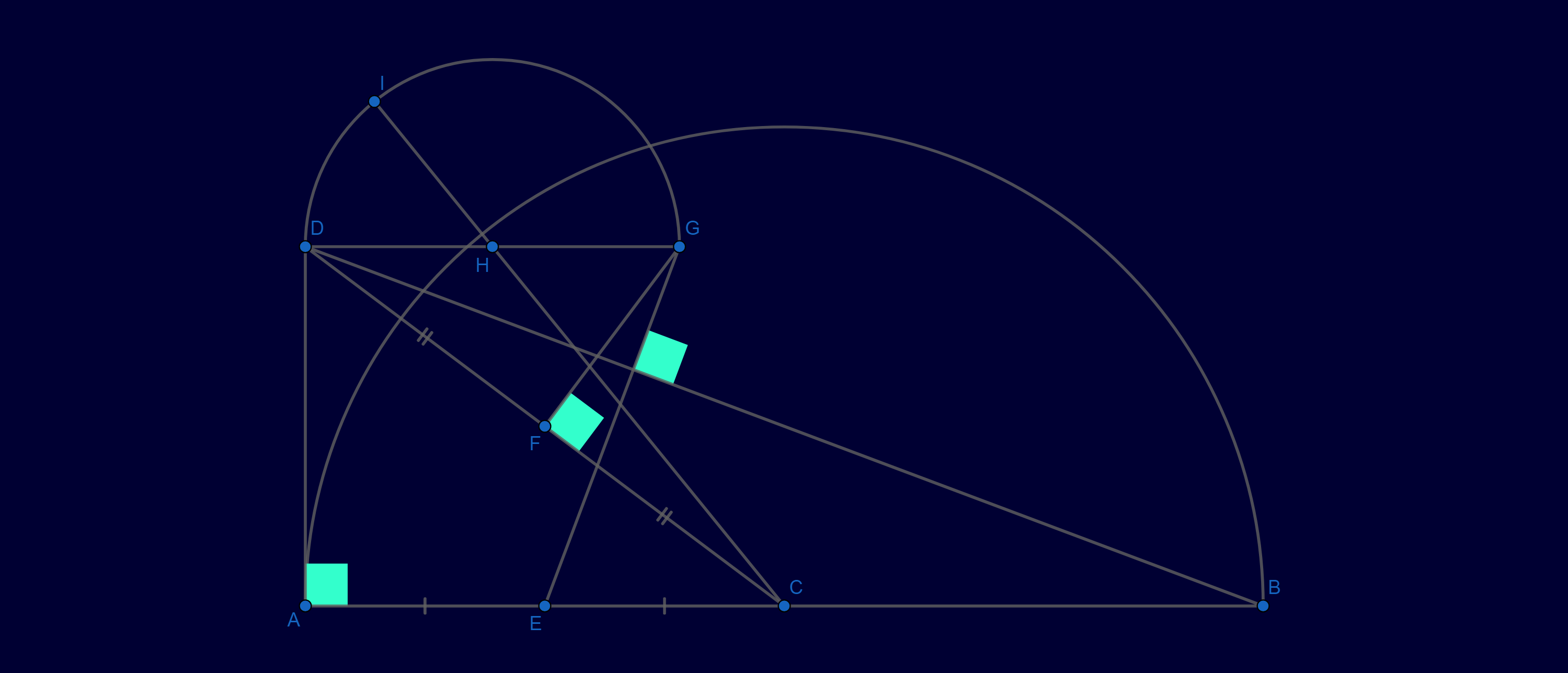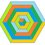# Homework is given to a child who is not ready for a math competition...

$A$ and $B$ are fixed points. Determine the locus of point $I$ as $D$ moves on the perpendicular from $A$ to the segment $AB$.Please help me!Note by Thành Đạt Lê
1 year, 10 months ago

This discussion board is a place to discuss our Daily Challenges and the math and science related to those challenges. Explanations are more than just a solution — they should explain the steps and thinking strategies that you used to obtain the solution. Comments should further the discussion of math and science.

When posting on Brilliant:

• Use the emojis to react to an explanation, whether you're congratulating a job well done , or just really confused .
• Ask specific questions about the challenge or the steps in somebody's explanation. Well-posed questions can add a lot to the discussion, but posting "I don't understand!" doesn't help anyone.
• Try to contribute something new to the discussion, whether it is an extension, generalization or other idea related to the challenge.

MarkdownAppears as
*italics* or _italics_ italics
**bold** or __bold__ bold
- bulleted- list
• bulleted
• list
1. numbered2. list
1. numbered
2. list
Note: you must add a full line of space before and after lists for them to show up correctly
paragraph 1paragraph 2

paragraph 1

paragraph 2

[example link](https://brilliant.org)example link
> This is a quote
This is a quote
    # I indented these lines
# 4 spaces, and now they show
# up as a code block.

print "hello world"
# I indented these lines
# 4 spaces, and now they show
# up as a code block.

print "hello world"
MathAppears as
Remember to wrap math in $$ ... $$ or $ ... $ to ensure proper formatting.
2 \times 3 $2 \times 3$
2^{34} $2^{34}$
a_{i-1} $a_{i-1}$
\frac{2}{3} $\frac{2}{3}$
\sqrt{2} $\sqrt{2}$
\sum_{i=1}^3 $\sum_{i=1}^3$
\sin \theta $\sin \theta$
\boxed{123} $\boxed{123}$

Sort by:

Are the points C and E also fixed???? Because, if they are, then how do you determine the position of G??? And is H the midpoint of DG???

- 1 year, 10 months ago

$C$, $E$ and $H$ are respectively the midpoints of $AB$, $AC$, $DG$.

- 1 year, 10 months ago

Okay.....but how do we define G???

- 1 year, 10 months ago

Is it the intersection of perpendicular bisector of DC and the line perpendicular to DB passing through E???

- 1 year, 10 months ago

Yup, it is.

- 1 year, 10 months ago

Ohhkay........coz, the picture was a bit misleading.....I assumed that DG is always parallel to AB, which isn't the case........

- 1 year, 10 months ago

You can prove that.

- 1 year, 10 months ago

Umm nope..........that isn't happening always........see my comment and my approach....you'll see what I mean

- 1 year, 10 months ago

But, I think the method I am using is lengthy...........What I am doing is, I have set up the Coordinate plane with the origin at C, then points A and B are (-2a,0) and (2a,0) and so, the point E becomes (-a,0)..........Now, we can give D the co-ordinates (-2a, 2y).........(Here, a is a real positive number, and y ranges.....).....Now, after some messy equation solving, I have the co-ordinates of G and H..........Here I have another doubt........Is I lying on the circle with DG as a diameter???

- 1 year, 10 months ago

Try to solve the problem. I already know the answer is a combination of half-parabola and a semicircle. But I don't know anything more specific. And $DG$ is a diameter.

- 1 year, 10 months ago